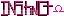-=+=- -=+=- -=+=- -=+=- -=+=- -=+=- -=+=- -=+=- -=+=- -=+=- -=+=- -=+=- -=+=- -=+=- -=+=- -=+=- -=+=- -=+=- -=+=- -=+=- -=+=- -=+=- -=+=- -=+=- -=+=- -=+=- -=+=- -=+=- -=+=- -=+=- (c) WidthPadding Industries 1987 0|151|0 -=+=- -=+=- -=+=- -=+=- -=+=- -=+=- -=+=- -=+=- -=+=- -=+=- -=+=- -=+=- -=+=- -=+=- -=+=- -=+=- -=+=- -=+=- -=+=- -=+=- -=+=- -=+=- -=+=- -=+=- -=+=- -=+=- -=+=- -=+=- -=+=- -=+=-
SoCoder -> Article Home -> Advanced Techniques

SchererererCreated : 08 February 2008
Edited : 24 March 2009
Language : C/C++

### C# Tips and Tricks

Tricks You Might Have Never Heard Of

Tricks You Might Have Never Heard Of
-------------------------------------------

We all like to learn little tricks and things to make our lives easier. This articles lists some of the little things i've learned over the years. This article mainly applies to C#, but also still affects C, C++, and Java in places where listed.

the '?' operator::.
C, C++, C#, Java
This operator can be used to do short-hand if...else coding on a single line. This is useful for making things less verbose or otherwise faster coding/less lines of coding. Let's take one example, where we want to assign a variable x to equal 5 if y is less than or equal to 5, and equal y if it is greater than 5. In a normal situation, the code would look like this:

However! I would like to save some space! Instead, I can write the same logic a different way to make it look like this:

as you can see, that was a major reduction in code clutter! Syntax for the operation looks like this:

the '??' operator ::.
C#
this piece works similarly to the last one, as being a short-hand conditional, but only in a specific scenario. The syntax for it is:

The way it works is, if <var1> is not null, it puts <var1> into <variable>; if it is null, however, it uses <var2>

the 'as' operator ::.
C#
We all know about typcasting when it comes to OOP, as it is used frequently. 'as' does the same exact thing, except instead of throwing an exception when it can't cast the object, it just returns a null value. It works like this:

the 'is' operator ::.
C#, Java*
This operator is used to check if an object is compatible/castable as some class. It is used like this:

* For Java, use the instanceof operator instead of is

the ',' operator ::.
C, C++, C#, Java

get more out of your enum ::.
C#
enum is a cool way to make custom understandable/readable sets of constants. What you may not know, is that you can make some values of enum encompass others, such as in this scenario:

String vs. string || int vs. Int32 || object vs. Object || ...etc ::.
C#
String is the same thing as string. int and Int32 are the same (on 32 bit platforms). object and Object are the same. Some people use Int32/Int64 instead of int to make a distinct differentiation between the two classes when they have multiple versions of a program. But other than that, they're the same.

Saturday, 09 February 2008, 02:56
JL235So how is String different to string? Is one an object and the other an array of chars? I've always wondered. Plus Java has enums too.
Saturday, 09 February 2008, 03:28
PhoenixUseful, Instinct! I didn't know abot the ?? operator.
Saturday, 09 February 2008, 05:38
JL235Ruby has similar, you can just use ||, but I've rarely found it as useful as you'd think.
Saturday, 09 February 2008, 09:08
SchererererString is the exact same thing as string. The keyword string is just a "shadow copy" of String. They both reference the same class and are interchangable.Tuesday, 24 March 2009, 11:37
PhoenixExtension Methods
Using the 'this' prefix on function arguments, you can add additional member functions to already existing classes, as shown here.

Lambda Expressions
These are very powerful, and were added in C# 3.0. Basically, they allow you to create tiny functions without typing out the whole thing.

LINQ
Related to the Lambda Expressions. Very SQL-like queries which can be used to filter data. Many examples here.